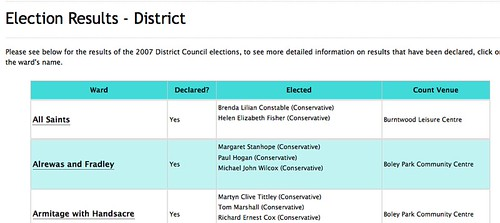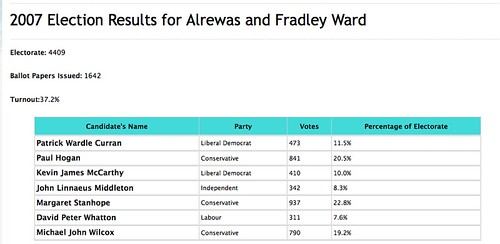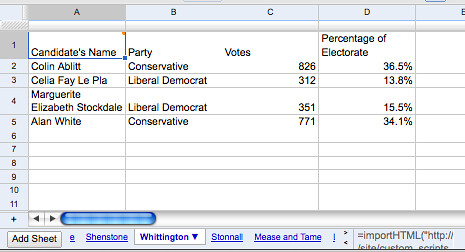Exciting news: Google Apps script is now available for all Google spreadsheet users…, so it seems I was timely in starting to get to grips with playing with this stuff…;-)

So what can we do with Google Apps Script? I’ve been posting a few ideas already, but here’s something I was working on last night – a script that automates table screenscraping using the Google spreadsheet =importHTML() formula.

Here’s the setting: Lichfield council lists details of the last round of council elections on a summary results page:and a set of individual pages detailing comprehensive results from each ward (example).What I wanted was a big table listing all the results by ward.

It’s easy enough to pull these results into a Google Spreadsheet using the =importHTML(“URL”,”table”,N) formula (where N is the number of the table in the page), but pulling results in for all 26 wards and then processing them separately would be laborious, so here’s what I did…

First of all, pull in the summary table to get a list of all the wards:The results by ward page all live on a commonly structured URL (e.g. http://www.lichfielddc.gov.uk/site/custom_scripts/electiondetail.php?ward=2&category=1) with the ward parameter identifying the ward. This URL isn’t picked up by the table scraper, so I had to do a bit of hand finishing, adding an ID column to which I added the appropriate ward number of each ward as used in the ward results URLs.

So, having got a sheet that listed the wards, and a (hand added) identifier for each ward, I could write a script that would create a separate sheet for each ward and add an appropriately customised =importHTML() formula to it:

```function addSheets() {

var sheet = ss.getSheetByName("Wards");
var wardNameID=[];
var wardIDName=[];
if (sheet != null) {
var col_ward=getColNumber(sheet,"Ward");
var col_ID=getColNumber(sheet,"ID");
var startRow = 2;  // First row of data to process
var numRows = getMaxRows(sheet);
var maxcols= getMaxColumns(sheet);

var dataRange = sheet.getRange(startRow, 1, numRows, maxcols);
var data = dataRange.getValues();
for (i in data) {
var row = data[i];
var wardName=row[col_ward];
var wardID=row[col_ID];
//Browser.msgBox(wardName+" "+wardID);
wardNameID[wardName]=wardID;
wardIDName[wardID]=wardName;
}
}
for (var i=2;i<numRows;i++){//28
var sname=wardIDName[i];
ss.insertSheet(sname, 1);
sheet = ss.getSheetByName(sname);
}
}```

This function uses a couple of utility functions – getMaxRows() and getMacColumns() which I superstitiously added to get the number of populated rows/columns from a sheet:

```function getMaxRows(sheet){
var maxrows = sheet.getMaxRows();var max =maxrows;
for (var j=1;j<maxrows+1;j++){
var header= sheet.getRange(j, 1, 1, 1).getValue();
max=j; j=maxrows+1;
}
}
return max;
}

function getMaxColumns(sheet){
var maxcols=sheet.getMaxColumns(); var max=maxcols;
for (var j=1;j<maxcols+1;j++){
var header= sheet.getRange(1, j, 1, 1).getValue();
max=j; j=maxcols+1;
}
}
return max;
}```

and the more generally useful getColNumber(), which gets the number of a column in the sheet given its header:

```function getColNumber(sheet,colName){
var colNum=-1;
var maxcols=sheet.getMaxColumns();
for (j=1;j<maxcols+1;j++){
var header= sheet.getRange(1, j, 1, 1).getValue();
}
return colNum;
}```

The addTableImporter() function is the one that adds the importTable() formula to each sheet as required:

```function addTableImporter(ss,n) {
var sc = ss.getActiveCell();

var arr = [];
var c = [];
c='=importHTML("http://www.lichfielddc.gov.uk/site/custom_scripts/electiondetail.php?ward='+n+'&category=1","table",1)';;
arr=c;

var destinationRange = ss.getRange(1 , 1, 1,1);

destinationRange.setValues(arr);
}```

Running the addSheets() function creates one sheet per ward, imports the appropriate table, and names the sheet as the name of the Ward.We’re now in a position to pull together a monolithic table that aggregates data from all the wards:

```function aggregator(){
var table=[];
table.push(["Ward","Candidate","Party","Vote"]);
var writeRow=[];
var bigsheet = ss.getSheetByName("Aggregate");
var wards=getWardNames();
for (var i=0;i<wards.length;i++){
//get sheet
var sheet = ss.getSheetByName(wards[i]);
if (sheet!=null){
var maxrows=getMaxRows(sheet);
for (var j=2;j<maxrows+1;j++){
var candidate=sheet.getRange(j, 1, 1, 1).getValue();
var party=sheet.getRange(j, 2, 1, 1).getValue();
var vote = sheet.getRange(j, 3, 1, 1).getValue(); if (typeof vote != 'number') vote=100;
if (candidate!=""){
writeRow=[wards[i],candidate,party,vote];
table.push(writeRow);
}
}
}
}
var destinationRange = bigsheet.getRange(1, 1, table.length, 4);
destinationRange.setValues(table);
}```

Again, I make use of a utility function, this time to grab the names of the wards from the ward spreadsheet:

```function getWardNames(){

var sheet = ss.getSheetByName("Wards");
var wardNames=[];
if (sheet != null) {
var col_ward=getColNumber(sheet,"Ward");
var startRow = 2;  // First row of data to process
var numRows = getMaxRows(sheet);
var dataRange = sheet.getRange(startRow, 1, numRows, 1);
var data = dataRange.getValues();
for (i in data) {
var row = data[i];
wardNames[wardNames.length]=row[col_ward];
}
}
return wardNames;
}```

Running the aggregator function pulls all the data into one sheet:Finally, for completeness, I added a routine that will delete the separate ward sheets:

```function deleteWardSheets(){
var wardNames=getWardNames();
for (var i=0;i<wardNames.length;i++){
ss.setActiveSheet(ss.getSheetByName(wardNames[i]));
ss.deleteActiveSheet();
}
}```

Supposedly, it’s possible to share scripts by submitting them to an Apps script gallery:On submitting a script, it appears to get passed into an approvals process, so I’m not sure what happens next…?

Anyway – the code is all provided above if you want to try it out. Remember, what it’s doing is looking at a list of Ward names and identifiers, creating a sheet for each Lichfield local council ward, importing the election results table for each ward from the Lichfield site into the appropriate sheet, then providing another function that generates an aggregated datatable containing all the results, annotated with the name of the appropriate ward.

I was wondering whether this could all be done with from single function call, but I got twitchy that if I called a sheet before the imported data was loaded everything would break.

The take home from me about this is that with a little bit of creativity you can mix and match spreadsheet formula and Javascript functions. (I don’t know if spreadsheet formula can be accessed directly from the apps script?) That is, you can run calculations either in Javascript/Apps script, or if it’s more convenient to use a spreadsheet formula, you can…

## Author: Tony Hirst

I'm a Senior Lecturer at The Open University, with an interest in #opendata policy and practice, as well as general web tinkering...

## 5 thoughts on “Screenscraping With Google Spreadsheets App Script and the =importHTML Formula”

1. Hi Tony:
I really like what you have done. However, I run into “You do not have permission to call setValues” error, when I try to screenscrape from http://finance.yahoo.com/q/op?s=GOOG for instance. (In your example, you have used http://www.lichfielddc.gov.uk/site/custom_scripts/electiondetail.php?ward=2&category=1 as the source).